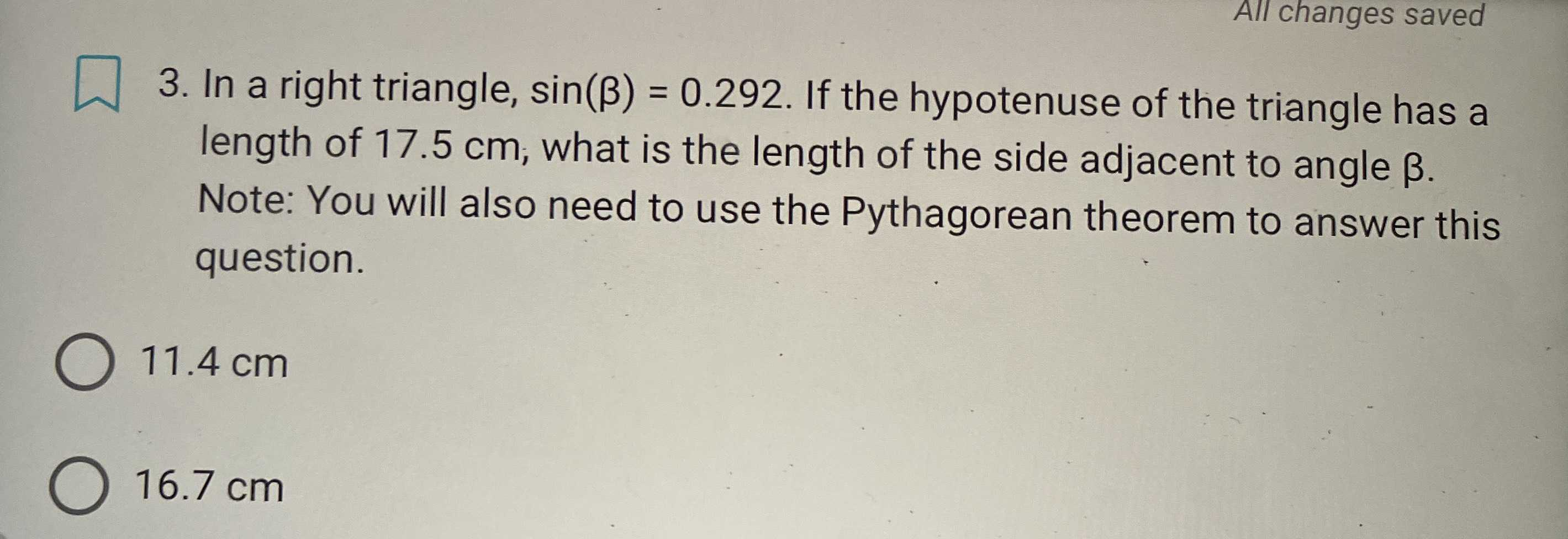### Still have math questions?

Trigonometry
QuestionIn a right triangle, $$\sin ( \beta ) = 0.292$$ . If the hypotenuse of the triangle has a length of $$17.5 cm$$ ; what is the length of the side adjacent to angle $$\beta$$ . Note: You will also need to use the Pythagorean theorem to answer this question.

$$11.4 cm$$

$$16.7 cm$$

$$16.7 cm$$